## DEFISHEYE

 Corrects for fisheye distortion in an image.

 USAGE: defisheye [-i ifov] [-o ofov] [-t type] [-f format] [-c xc,yc] [-r radius] [-a angle] infile outfile USAGE: defisheye -d [-c xc,yc] [-r radius] [-s strokecolor] infile outfile USAGE: defisheye [-h or -help] -i .... ifov ......... input (fisheye) image field of view in degrees; ...................... float; 00; default=min(width,height)/2 -a .... angle ........ angle of rotation (clockwise) for the perspective image; ...................... float; 0<=angle<360; default=0 -d ................... draw circle on the input fisheye image of specified radius, ...................... center and color=scolor, which will then become the output -s .... scolor ....... scolor is the stroke color for a circle that will be ...................... drawn on the input fisheye image; default=white PURPOSE: To correct for fisheye distortion in an image. DESCRIPTION: DEFISHEYE is designed to transform a fisheye image into a normal perspective view looking towards the center of the fisheye image. ARGUMENTS: -i ifov ... IFOV is the input fisheye image field of view in degrees. A value of 180 will correspond to a hemispherical fisheye image within the circular area. Values are floats in the range 0

### EXAMPLES

 Mandril (Square) Image - Artificially Created Fisheye Types / Circular (Created With FISHEYE Script: Perspective FOV=120; Fisheye FOV=180) Fisheye Distorted Images Linear Equal Area Orthographic Stereographicoriginal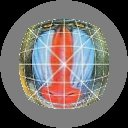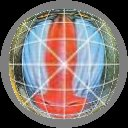Corrected Images Linear Arguments: -o 120 -i 180 -t linear -f circular Equal Area Arguments: -o 120 -i 180 -t equalarea -f circular Orthographic Arguments: -o 120 -i 180 -t orthographic -f circular Stereographic Arguments: -o 120 -i 180 -t stereographic -f circularoriginal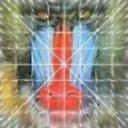Woman (Non-Square Image) - Artificially Created Lineaer Fisheye - Circular vs. Fullframe (Created With FISHEYE Script: Perspective FOV=120; Fisheye FOV=180) Fisheye Distorted Images Linear Circular Linear Full FrameoriginalCorrected Images Linear Circular Arguments: -o 120 -i 180 -t linear -f circular Linear Full Frame Arguments: -o 120 -i 180 -t linear -f fullframeoriginal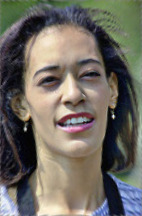Computer Generated Linear Circular Fisheye Image - Correct vs Incorrect Type http://www.path.unimelb.edu.au/~bernardk/tutorials/360/background/projections.html http://wiki.panotools.org/Fisheye_Projection http://wiki.panotools.org/Projections Original Linear Arguments: -o 120 -i 180 -t linear -f circular Equal Area Arguments: -o 120 -i 180 -t equalarea -f circular Orthographic Arguments: -o 120 -i 180 -t orthographic -f circular Stereographic Arguments: -o 120 -i 180 -t stereographic -f circular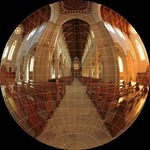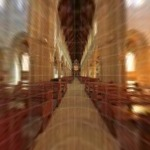Computer Generated Linear Circular Fisheye Image (Full Size) http://www.path.unimelb.edu.au/~bernardk/tutorials/360/background/projections.html http://wiki.panotools.org/Fisheye_Projection http://wiki.panotools.org/Projections Original Linear Arguments: -o 120 -i 180 -t linear -f circular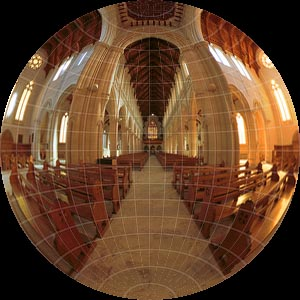Linear Full Frame Fisheye Image http://www.all-in-one.ee/~dersch/perspective/Wide_Angle_Perspective.html Original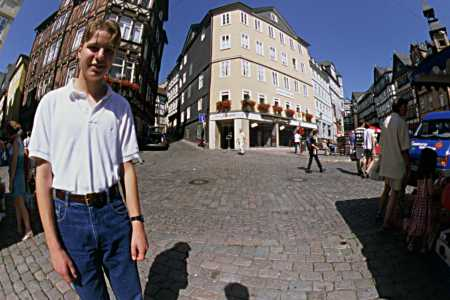Corrected Arguments: -o 120 -i 180 -t linear -f circular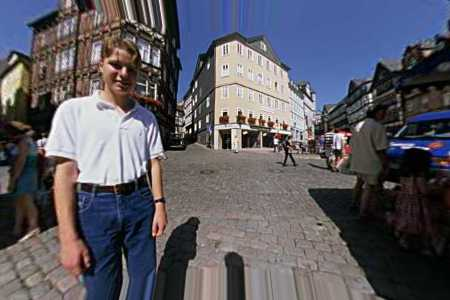TrimmedEquisolid Full Frame Fisheye Image http://en.wikipedia.org/wiki/Fisheye_lens OriginalCorrected Arguments: -o 120 -i 170 -t linear -f circularPerspective Correctedand Trimmed 3Drotate tilt=30 auto=outLinear Circular Fisheye Image - Adjustments http://www.worldserver.com/turk/quicktimevr/defish/defish.html Original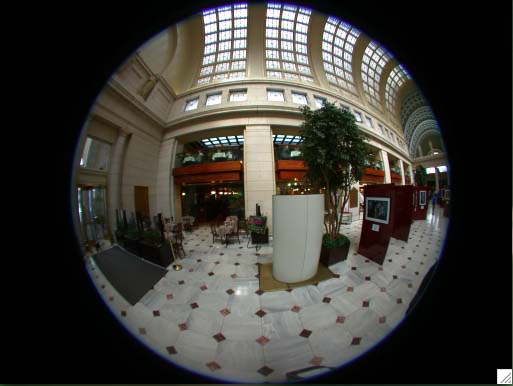Original With Default Circle Arguments: -d [-r 193 -c 256,192.5]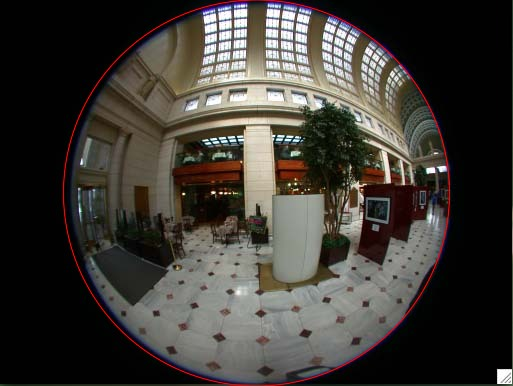Original With Adjusted Circle Arguments: -d -r 190 -c 260,192.5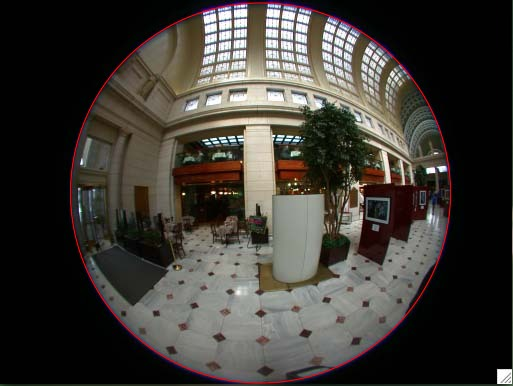Under Corrected Outward Bowing Arguments: -o 120 -i 180 -t linear -f circular -r 190 -c 260,192.5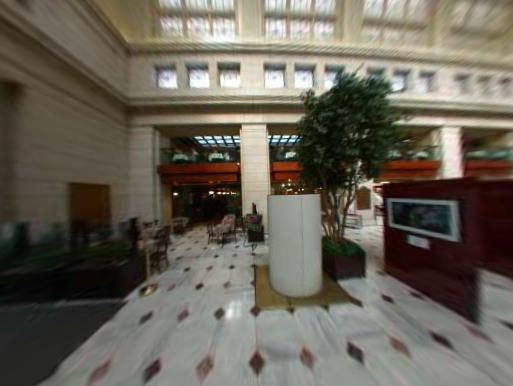Over Corrected Inward Bowing Arguments: -o 120 -i 190 -t linear -f circular -r 190 -c 260,192.5Good Correction Straight Edges Arguments: -o 120 -i 188 -t linear -f circular -r 190 -c 260,192.5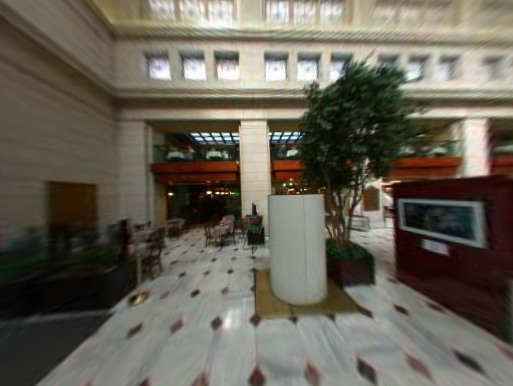What the script does is as follows: For a linear circular fisheye distortion, it applies the following equations: perspective: r=f*tan(phi); f=(N/2)/tan((fov/2)*(pi/180)) fisheye: r=f*phi; f=(N/2)/((fov/2)*(pi/180)) where N=smaller dimension This is equivalent to the following IM commands for the case of a linear circular fisheye image of \${dim}x\${dim} to perspective image: compute image dimensions and center coordinates, etc xd="xd=i-\$xc;" yd="yd=j-\$yc;" rd="rd=hypot(xd,yd);" phi="phi=atan(\$ofocinv*rd);" ifoc=`convert xc: -format "%[fx:(\$dim*180)/(\$ifov*pi)]" info:` rr="rr=\$ifoc*phi;" xs="xs=(rd?rr/rd:0)*xd+\$xc;" ys="ys=(rd?rr/rd:0)*yd+\$yc;" convert \$infile -virtual-pixel black -fx \ "\$xd \$yd \$rd \$phi \$rr \$xs \$ys u.p{xs,ys}" \ \$outfile